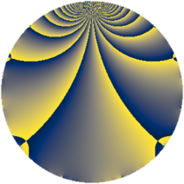# Properties

 Label 1323.2.hLevel $1323$ Weight $2$ Character orbit 1323.h Rep. character $\chi_{1323}(226,\cdot)$ Character field $\Q(\zeta_{3})$ Dimension $72$ Newform subspaces $8$ Sturm bound $336$ Trace bound $5$

# Related objects

## Defining parameters

 Level: $$N$$ $$=$$ $$1323 = 3^{3} \cdot 7^{2}$$ Weight: $$k$$ $$=$$ $$2$$ Character orbit: $$[\chi]$$ $$=$$ 1323.h (of order $$3$$ and degree $$2$$) Character conductor: $$\operatorname{cond}(\chi)$$ $$=$$ $$63$$ Character field: $$\Q(\zeta_{3})$$ Newform subspaces: $$8$$ Sturm bound: $$336$$ Trace bound: $$5$$ Distinguishing $$T_p$$: $$2$$, $$5$$

## Dimensions

The following table gives the dimensions of various subspaces of $$M_{2}(1323, [\chi])$$.

Total New Old
Modular forms 384 88 296
Cusp forms 288 72 216
Eisenstein series 96 16 80

## Trace form

 $$72q - 2q^{2} + 66q^{4} + 5q^{5} + 12q^{8} + O(q^{10})$$ $$72q - 2q^{2} + 66q^{4} + 5q^{5} + 12q^{8} + 6q^{10} - 3q^{11} + 3q^{13} + 54q^{16} + 9q^{17} + 4q^{20} - 4q^{23} - 21q^{25} + 16q^{26} + 18q^{29} - 6q^{31} + 82q^{32} + 3q^{37} + 19q^{38} + 6q^{40} + 10q^{41} + 11q^{44} - 12q^{46} - 54q^{47} + 45q^{50} + 15q^{52} - 16q^{53} + 6q^{55} + 9q^{58} - 60q^{59} - 12q^{62} + 12q^{64} + 60q^{65} - 12q^{67} + 30q^{68} - 6q^{71} - 12q^{73} - 41q^{74} - 6q^{76} - 36q^{79} + 19q^{80} + 18q^{83} + 3q^{85} - 25q^{86} - 9q^{88} + 41q^{89} + 52q^{92} - 6q^{94} - 34q^{95} + 3q^{97} + O(q^{100})$$

## Decomposition of $$S_{2}^{\mathrm{new}}(1323, [\chi])$$ into newform subspaces

Label Dim. $$A$$ Field CM Traces $q$-expansion
$$a_2$$ $$a_3$$ $$a_5$$ $$a_7$$
1323.2.h.a $$2$$ $$10.564$$ $$\Q(\sqrt{-3})$$ None $$-2$$ $$0$$ $$1$$ $$0$$ $$q-q^{2}-q^{4}+(1-\zeta_{6})q^{5}+3q^{8}+(-1+\cdots)q^{10}+\cdots$$
1323.2.h.b $$6$$ $$10.564$$ $$\Q(\zeta_{18})$$ None $$-6$$ $$0$$ $$-3$$ $$0$$ $$q+(-1+\zeta_{18}^{3}+\zeta_{18}^{4})q^{2}+(1-\zeta_{18}^{3}+\cdots)q^{4}+\cdots$$
1323.2.h.c $$6$$ $$10.564$$ $$\Q(\zeta_{18})$$ None $$-6$$ $$0$$ $$3$$ $$0$$ $$q+(-1+\zeta_{18}^{3}+\zeta_{18}^{4})q^{2}+(1-\zeta_{18}^{3}+\cdots)q^{4}+\cdots$$
1323.2.h.d $$6$$ $$10.564$$ 6.0.309123.1 None $$2$$ $$0$$ $$-5$$ $$0$$ $$q-\beta _{1}q^{2}+(1-\beta _{1}+\beta _{3})q^{4}+(-\beta _{2}+\cdots)q^{5}+\cdots$$
1323.2.h.e $$6$$ $$10.564$$ 6.0.309123.1 None $$2$$ $$0$$ $$5$$ $$0$$ $$q-\beta _{1}q^{2}+(1-\beta _{1}+\beta _{3})q^{4}+(\beta _{2}+\beta _{3}+\cdots)q^{5}+\cdots$$
1323.2.h.f $$10$$ $$10.564$$ 10.0.$$\cdots$$.1 None $$4$$ $$0$$ $$4$$ $$0$$ $$q+(\beta _{1}-\beta _{5})q^{2}+(1+\beta _{3})q^{4}+(-\beta _{6}+\cdots)q^{5}+\cdots$$
1323.2.h.g $$12$$ $$10.564$$ $$\mathbb{Q}[x]/(x^{12} - \cdots)$$ None $$-4$$ $$0$$ $$0$$ $$0$$ $$q-\beta _{1}q^{2}+(1+\beta _{1}+\beta _{5})q^{4}+\beta _{2}q^{5}+\cdots$$
1323.2.h.h $$24$$ $$10.564$$ None $$8$$ $$0$$ $$0$$ $$0$$

## Decomposition of $$S_{2}^{\mathrm{old}}(1323, [\chi])$$ into lower level spaces

$$S_{2}^{\mathrm{old}}(1323, [\chi]) \cong$$ $$S_{2}^{\mathrm{new}}(63, [\chi])$$$$^{\oplus 4}$$$$\oplus$$$$S_{2}^{\mathrm{new}}(189, [\chi])$$$$^{\oplus 2}$$$$\oplus$$$$S_{2}^{\mathrm{new}}(441, [\chi])$$$$^{\oplus 2}$$